or# Triangles NCERT Solutions : Class 9 Maths

## NCERT Solutions for Class 9 Maths : TrianglesFilter
Filters :
Classes
•
•
•
•
•
•
•
Chapters
•
•
•
•
•
•
•
•
•
•
• 7 More
Exercises
•
•
•
•
•
•

Doubtnut one of the best online education platform provides free NCERT Solutions of Maths for Class 9 Triangles which are solved by our maths experts as per the NCERT (CBSE) guidelines. We provide the solutions as video solutions in which concepts are also explained along with answer which will help students while learning , preparing for exams and in doing homework. These Solutions will help to revise complete syllabus and score more marks in examinations.

Get here free, accurate and comprehensive NCERT Solutions for Class 9 Maths Triangles which have been reviewed by our maths counsellors as per the latest edition following up the CBSE guidelines. We provide video solutions in which solutions to all the questions of NCERT Class 9 Maths textbook are explained in step by step and detailed way.

## The Topics of the Chapter Triangles are :

SUFFICIENT CONDITION FOR CONGRUENCE OF TRIANGLE

EXTERIOR ANGLE OF A TRIANGLE

PROPERTIES OF A TRIANGLE

CONGRUENCE

SOME INEQUALITY RELATION IN A TRIANGLE

CONGRUENCE OF LINE SEGMENTS

It contains these exercises along with solved examples. All the exercises are solved in the video. Select the exercise to view the solutions exercisewise of the Chapter Triangles:

## NCERT Solutions Class 9 Maths Chapter Triangles Exercises:

We have covered all the exercises and also Solved examples in the videos. Along with the practise exercise Students should also practise solved examples to clear the concepts of the Triangles. If incase you have any doubt you can watch the solutions for the given questions.Watch the solutions for the given questions in which it is also explained in the video steps to solve the questions along with answers.

Until now, students must be familiar with different shapes and their major properties like rectangles, squares, circles, triangles, etc. In this lesson, we will primarily focus on Triangles and every theorem, laws, and properties of it in detail. The NCERT textbook provides the perfect amount of information needed for the students. Thus, it is the best and important for students to go through it.

When talking about doing great in academics, children make all the required efforts to get the best study material as they get confused as to what to study, how to study and from where to study. Due to this confusion, they end up wasting their time on wrong elaborated material, which will take much of their time and don’t get to study the exact thing. Therefore, when searching for the best material why to run here and there when the best material is available in the market in the best price ever, which is not hard on anyone’s pocket. Students must go for NCERT solutions always as it is undefeated by any means from the plenteous options stalked in the market.

It is best for the CBSE Students because each and every topic is covered by the NCERT textbooks from basic to tedious concepts.

Moreover, Doubtnut, an online learning platform provides the explanation of the concept and solutions to the problems of the NCERT class 9 books in the most innovative manner so that learning becomes easier for the students. It provides video-tutorials and graphical representation wherever required to make the concepts understandable in an easy language.

Keeping in mind the phobia that children develop regarding a topic or a subject as a whole, Doubtnut.com provides the study material in a way that the student do not get bored, yet learns, and reads the topics covered in syllabus in an interesting way. We try to explain each and every concept through diagrams, graphs, and pictures and provide some related questions for practice so that when child completes the chapter readings, he is ready to solve any question related to the topic and practice it whenever needed.

Therefore, to study in a fun way student must download our app to go through the chapters and make learning easy and fun.

## TRIANGLE

A polygon with three vertices and three edge.

Area of a triangle= ½ (length × base).

Perimeter =sum of side lengths of the triangle.

Note:

1. Sum of angles of any triangle is 180 degree.
2. For the equal sides of a triangle two dashes are drawn on the equal sides.

### TYPES OF TRIANGLES

#### Equilateral triangle

A triangle having three equal sides and angles. Having a 60 degrees angle on each corner.

#### Isosceles triangle

A triangle with two equal sides and two equal angles or with two acute angles and one obtuse angle.

#### Right-angled triangle

A triangle with one 90 degrees angle shown by a small square. It can be an isosceles triangle if other two sides are equal with 45 degrees angle each.

#### Scalene

A triangle having all of its angles different and none of its sides are same.

#### Obtuse

A triangle having three different angles with one of its angles greater than 90°. Having none of its sides equal in length.

Some examples to know what are we actually going to do in this chapter:

In the given figure F1 and F2-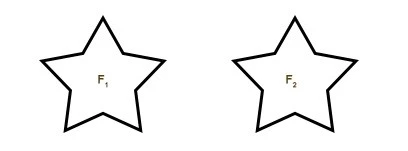We can see that they are mirror images hence they are congruent.

FI≈F2

Now, if we see in this case where we dealing with lines: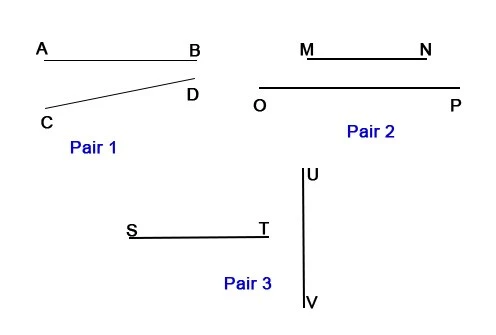Are they superimposable on each other, we observe:

AB≈CD

MN≠OP

ST≈UV

We observe that if two-line segments have equal lengths, they are congruent. Also, if two-line segments are congruent, they have equal lengths.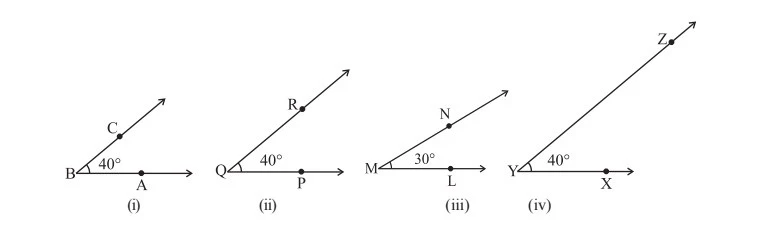Now if we look at different triangle-

1. If we try tracing them as before like âˆŸPQR on âˆŸABC, we observe that they are superimposable over each other. Thus, they are congruent and even the measure of congruent angles are same.

âˆŸPQR ≈âˆŸABC

1. Likewise tracing triangles LMN and ABC, they cannot be traced over each other as the line ML does not fall on line BC. Hence, they are not congruent.

âˆŸLMN ≠âˆŸABC

Let’s see âˆŸXYZ  and âˆŸABC, we see line YZ seems longer than line BC. Here that the rays in an angle only denotes the angle and not the length. Now on placing âˆŸXYZ on âˆŸABC we find that they are congruent.

âˆŸABC≈ âˆŸXYZ

CONCLUSION:

âˆŸABC≈ âˆŸPQR≈ âˆŸXYZ.

We observe that two angles of a triangle are congruent if they have same measures. So we can denote the congruency by = rather than≈.

## CRITERIA FOR CONGRUENCY OF TRIANGLES

Every time tracing and then coming to the conclusion of congruency is difficult. So there are some criterias:

Case 1. Trying to draw a congruent triangle to the given ABC we see that-

1. The length of all the three sides of ABC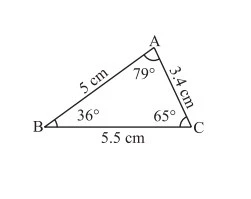OR

1. Length of two sides and angle between them

OR

1. â–²measures of two angles and length of the side included by them.

Drawing the triangle:

Length of line BC = 5.5cm

Length of line AC= 3.4 cm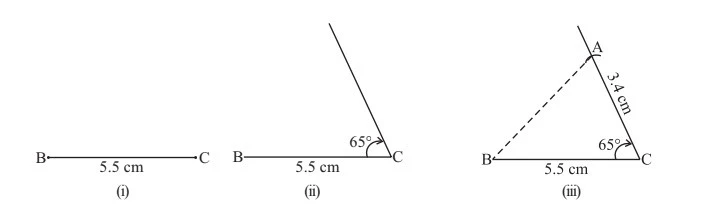We get âˆŸC = 65°.

Measuring the two angles and lengths of the side included by them is known.

Draw a triangle ABC

Length of line BC= 5.5 cm

âˆŸC = 65 degree

âˆŸB= 36 degree

»BC=5.5 cm drawing a line at 65 degree from point C and another line at 36 degree. The point of intersection is point A. Hence we list the criteria of congruency of triangles-

Like in the given figure Æ‰B = Æ‰Q and Æ‰C = Æ‰R and sides between Æ‰B and Æ‰C, Æ‰Q and Æ‰R are equal to each other therefore BC = QR,

â–²ABCâ–²PQR.

Like in the given figure Æ‰B = Æ‰Q and Æ‰C = Æ‰R and sides between Æ‰B and Æ‰C, Æ‰Q and Æ‰R are equal to each other therefore BC = QR,

â–²ABCâ–²PQR.

### THEOREM 1

1. SSS (Side-Side-Side)

When all the three sides of a triangle are equivalent corresponding to one another then the triangles are said to be congruent to each other following the SSS rule.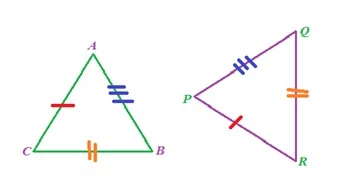In the given figure AC=PR and QR=BC and AB=PQ, hence ABC ≈PQR.

### THEOREM 2

1. SAS (Side-Angle-Side)

When two sides and an angle included between the sides of a given triangle is equivalent to the corresponding two sides and the angle between the sides of the second one then the triangles are said to be congruent to each other by SAS rule.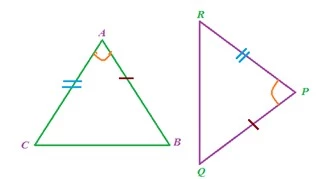In the given figure, the sides AC=PR, AB=PQ and the angle between AC and AB equal to angle between PR and PQ that is CA=RP, hence â–² ABC â–²PQR.

### THEOREM 3

1. ASA (Angle-Side-Angle)

When two angles and a side included between the angles of a triangle corresponds with two angles and a side included between the angles of the other triangle then the triangles are said to be congruent to each other by ASA rule.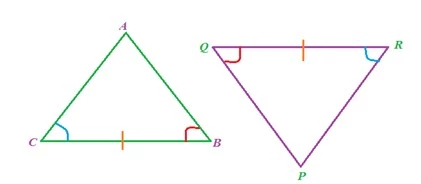Like in the given figure Æ‰B = Æ‰Q and Æ‰C = Æ‰R and sides between Æ‰B and Æ‰C, Æ‰Q and Æ‰R are equal to each other therefore BC = QR,

â–²ABCâ–²PQR.

Like in the given figure Æ‰B = Æ‰Q and Æ‰C = Æ‰R and sides between Æ‰B and Æ‰C, Æ‰Q and Æ‰R are equal to each other therefore BC = QR,

â–²ABCâ–²PQR

### THEOREM 4

1. RHS (Right Angle-Hypotenuse-Side)

When the hypotenuse and a side of a triangle is equivalent to the hypotenuse and side of the other triangle then both the sides are side to be congruent to each other.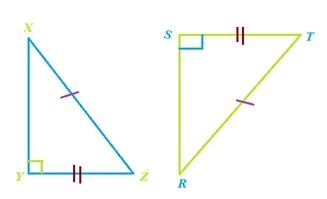Thus we can say that, in the above figure XZ =RT and side YZ= ST, Soâ–² XYZ â–²RST.

### THEOREM 5

1. AAS (Angle-Angle-Side):

When in the given triangles two angles and one non-included side of one triangle are equivalent corresponding to the other two angles and one-included side of the other triangle then the two triangles are said to be congruent by AAS rule.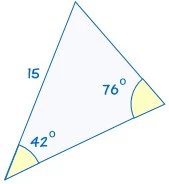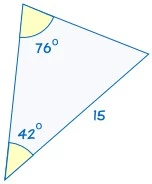Is congruent to-

NOTE: AAA means we are provided with all the three angles of the triangle, but we are not provided with any side so, we cannot drive any congruence between the triangle, as this is not enough information to drive any conclusion about congruence. Because triangles can have equal angles but be different in their sizes.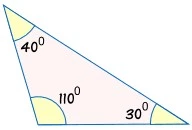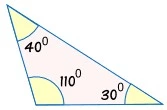So without knowing at least one of the sides we cannot tell the congruence between them.

INEQUALITY OF TRIANGLES

### VERTICAL ANGLES

Angle AOC and angle BOD are a pair of vertical angles if the line segments OA and OB are opposite rays of line AB and similarly if OC and OD are opposite rays of line CD.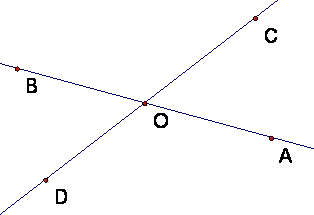THEOREM: In the given figure AOC and BOD are a pair of vertical angle, they are congruent.

PROOF: We can see that AOC and COB are supplementary. Their sum is 180°, similarly COB and BOD are supplementary.

### EXTERIOR ANGLE THEOREM

We can see in the given figure DAB is an exterior angle of the triangle ABC at A. There is another exterior angle at A formed by extending side AB in place of side AC, the two exterior angles are a pair of vertical angles.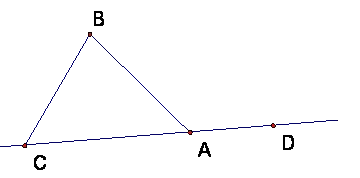THEOREM: An exterior angle at A of the triangle ABC is greater than either of the angles C or B.

PROOF: This can be explained by the figure: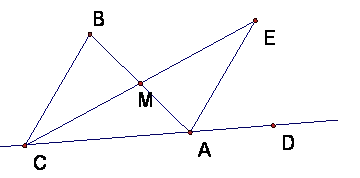M is the mid-point of AB and ME=CM so M is also the midpoint for CE.

So by using SAS rule these two â–² MBC = â–²MAE

âˆŸCMB =âˆŸEMA by vertical angles

MB=MA, M is mid-point of AB

CM=EM, M is mid-point of CE

âˆŸMBC=âˆŸMAE, but âˆŸMBC= âˆŸB in theâ–²

### GREATER ANGLE AND GREATER SIDE IN A TRIANGLE

THEOREM: In a â–²ABC, BC>CA, only if âˆŸCAB>âˆŸABC.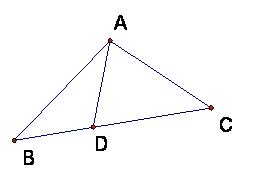A greater angle of a triangle is opposite a greater side. Let ABC is a triangle in which âˆŸABC> âˆŸBCA, then as a result side AC is also greater than side AB. If it is not greater, then AC is either equal to AB or less.

## Significance of NCERT Mathematics Book for Class 9

NCERT book covers each and every topic of the entire chapter in a detailed way.  It is must to read book before sitting for the examinations as it has everything you need to solve and various kinds of questions related to the topic. Therefore, it is important to have the NCERT book to score well. For further help regarding any concept or chapter or subject, you can always visit our website as it provides in-depth explanations of the concepts in a fun way. You can also just download our app to reach to us easily and we ensure that you will understand each and everything in a compiled manner if you look here.